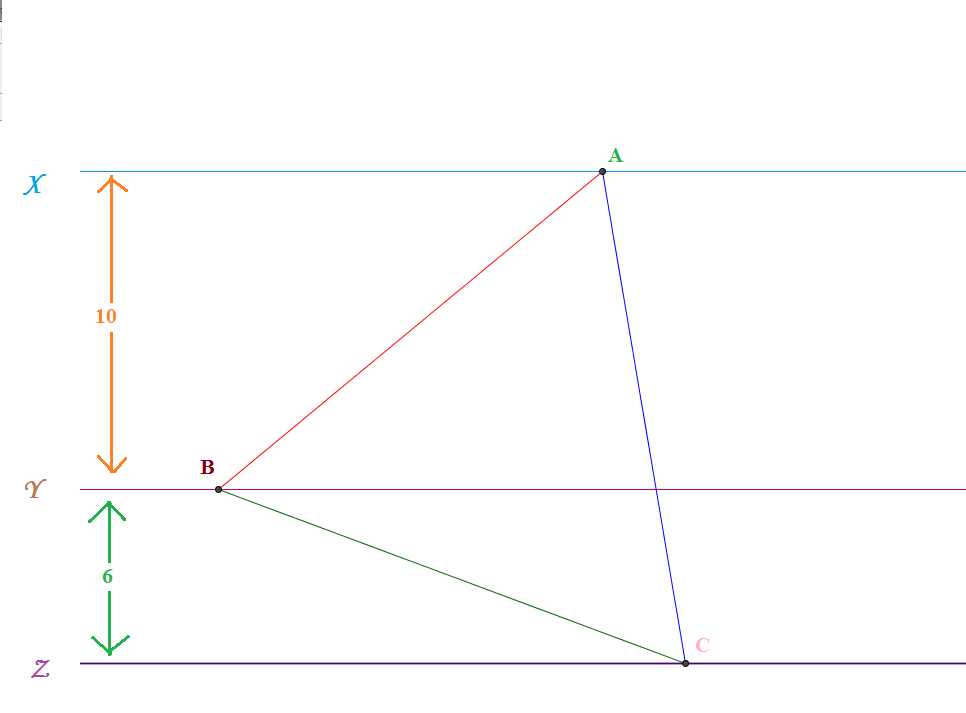# Tricky Triangle

Geometry Level 4$X$ , $Y$ and $Z$ are 3 mutually parallel straight lines in a plane, such that $Y$ is between $X$ and $Z$ and the distance between $X$ and $Y$ is $10$ units and the distance between $Y$ and $Z$ is $6$ units. $ABC$ is an equilateral triangle with $A$ on $X$ , $B$ on $Y$ and $C$ on $Z$. If the area of $\triangle ABC$ can be expressed as $\dfrac{a^2}{\sqrt{b}}$ where $a$ and $b$ are coprime positive integers and $b$ is not divisible by the square of any prime, find the value of $a+b$.

×